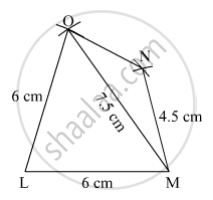SSC (English Medium) Class 8Maharashtra State Board
Share

# Construct the Following Quadrilateral of Given Measures. Construct ☐ Lmno Such that L(Lm) = L(Lo) = 6 Cm, L(On) = L(Nm) = 4.5 Cm, L(Om) = 7.5 Cm. - SSC (English Medium) Class 8 - Mathematics

#### Question

Construct the following quadrilateral of given measures.

Construct ☐ LMNO such that l(LM) = l(LO) = 6 cm, l(ON) = l(NM) = 4.5 cm, l(OM) = 7.5 cm.

#### Solution

Steps of Construction:

Step 1:
Draw LM = 6 cm.

Step 2: With L as centre and radius 6 cm, draw an arc.

Step 3: With M as centre and radius 7.5 cm, draw an arc cutting the previous arc at O.

Step 4: Join OL.

Step 5: With O as centre and radius 4.5 cm, draw an arc.

Step 6: With M as centre and radius 4.5 cm, draw an arc cutting the previous arc at N.

Step 7: Join ON and MN.Here, LMNO is the required quadrilateral.

Is there an error in this question or solution?

#### APPEARS IN

Balbharati Solution for Balbharati Class 8 Mathematics (2019 to Current)
Chapter 8: Quadrilateral : Constructions and Types
Practice Set 8.1 | Q: 4 | Page no. 43
Solution Construct the Following Quadrilateral of Given Measures. Construct ☐ Lmno Such that L(Lm) = L(Lo) = 6 Cm, L(On) = L(Nm) = 4.5 Cm, L(Om) = 7.5 Cm. Concept: Construction of a Quadrilateral.
S